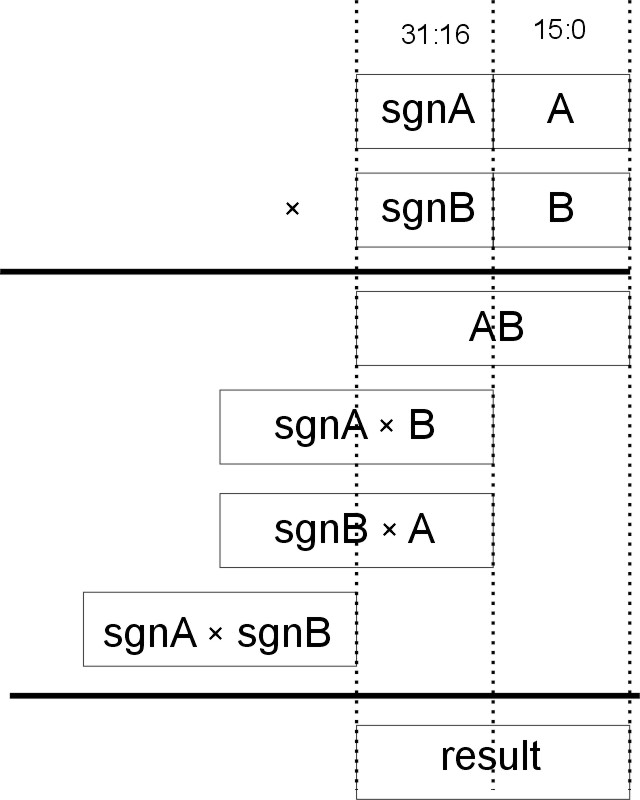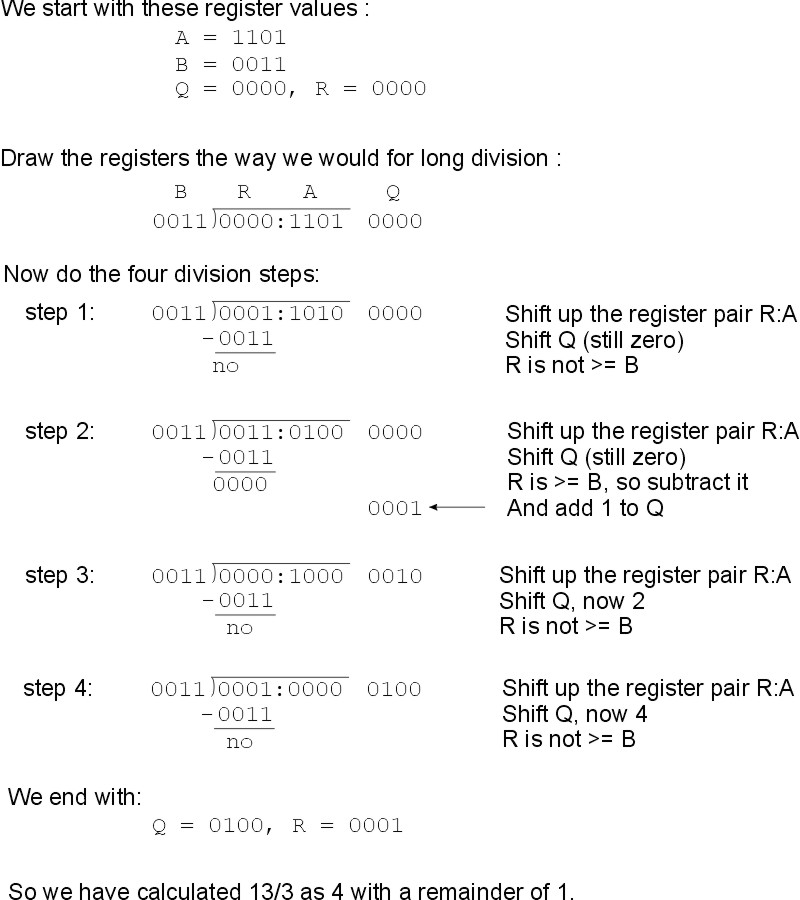Multiply, Division, Square Root
The multiplication, division and square root operations are implemented by iteration.

# Unsigned Multiplication

The algorithm the Megaprocessor uses is simple long hand multiplication. To multiply two n bit numbers A and B to give a 2n bit result:

initialise result to 0

for n steps

{

result = 2 * result

if most significant bit of B is 1

{

result = result + A

}

rotate left B

}

(In a rotation the register is shifted and the bit that "falls out" one end is put in at the other)

The main difficulty is that we need a 32 bit adder and accumulator to build up the result and the Megaprocessor is only 16 bit. To form the 32 bit result we use R2 and R3 together. There is a 32 bit shift register associated with R2 and R3 for this function (also used in division). To do the 32 bit addition we split it into two 16 bit parts. We use ADDER2 to carry out the LS 16 bits addition. Any carry from the LS 16 bit addition is stored in the X bit of the PS register. The MS 16 bits are added by ALU1 in the following cycle, using the carry bit from X as required. The algorithm is mapped onto the mgeaprocessor as follows:

we only calculate R0 × R1. The LS 16 bits of the result are stored in R2, and the MS 16 bits are stored in R3. R2 and R3 are initialised to 0.

there are 16 cycles of iteration. These are counted by the H register whilst the state machine is held in state 13

in each cycle of iteration:

ADD2 calculates the sum of left shifted R2 and either R0 if MSB of R1 is 1 otherwise zero.

R2 is set to the value calculated by ADD2. The carry out is stored in X.

ALU1 is set to calculate the sum of left shifted R3 and 2*X

R3 is set to the value calculated by ALU1.

R1 is rotated left

(We must use 2*X rather than just X as X represents the carry of an addition in the preceeding cycle, and we've shifted the data up by one place since then).

# Signed Multiplication

Signed multiplication builds on unsigned multiplication. If we look at multiplication using partial products when the 16 bit values A and B are sign extended to 32 bits we get :From this we can see that we can construct the result as follows:
LS 16 bits of result =  LS 16 bits of A × B (which we get from the unsigned multiplication of A B)
MS 16 bits of result = MS 16 bits of A × B(which we get from the unsigned multiplication of A B)
+ LS  16 bits of sgnA × B
+ LS  16 bits of sgnB × A

[The 16 bit sign extensions will be either all 0s (which is zero) or all 1s (which is -1).]

So to implement signed multiplication we first carry out an unsigned multiplication. Then we add an extra cycle to calculate the sum of those arguments that have the top (sign) bit set. This is stored in R0. Then in the finish cycle we subtract it from R3 (the MS 16 bits of the result) to change the result of the unsigned multiplication to be that of signed.

# Unsigned Division

I use the restoring division algorithm. This is described in various places e.g. Wikipedia. To map the algorithm into a shape the Megaprocessor could handle I found the form in "Computer Architecture A Quantitative Approach", Hennessy & Patterson particularly helpful. It is essentially the same as the long hand division we learnt when we were small.

To divide two n bit numbers A/B:

initialise two registers to 0, these will eventually hold the quotient and remainder so call them Q and R.
loop n times
{
shift Q up a bit
shift A up a bit and shift the output bit into R.
See if R is now bigger than or equal to B (the divisor).
If so then subtract B from R and add 1 to Q
}

The two registers R and A are being treated as a single 2n bit register. As an example consider dividing two 4 bit numbers 11012 / 00112 (13/3)....To do this using just the ALU would take several operations for each step. I've therefore added some extra resources to help speed it along. The algorithm is mapped onto the Megaprocessor as follows:

We can only calculate R0/R1.

R2 is used as register A and is initialised from R0 at the beginning of execution.

R1 is register B.

R3 is used as R. It is initialised to 0.

There is no explicit Q register. Instead we use the low order bits of R2. As we progressively shift R2 up into R3 we free up its low order bits and these are used for the quotient.

A 32 bit shift register is located with R2 and R3 to carry out the required shift (also used by the multiplication instruction). It input bit is determined by the result of the ALU.

The ALU is set to calculate RA - RB. RA is set to be the MSB of the 32 bit shift register, and RA is set to be R1. This is is equivalent to R - B.

Each cycle R2 is set to be the LSB of the 32 bit register. R3 takes the ALU result if it is positive (i.e R>B) or else the MSB of the shift register if not.

The 16 cycles of iteration are carried out with the state machine held in state 11, with the H register being used to count the steps.

The Megaprocessor therefore takes 18 cycles for unsigned division. 1 to initialise, 16 iteration cycles, and one to finish off.

# Signed division

This is implemented via unsigned division. There are two common ways of defining signed division. They differ as to whether or not negative remainders are allowed. Both are valid and both are defined. The mode is selected using the D flag of the PS register.

 D = 0 D = 1 13/3 Q = 4, R = 1 Q = 4, R = 1 13/-3 Q = -4, R = 1 Q = -4, R = 1 -13/3 Q = -4, R = -1 Q = -5, R = 2 -13/-3 Q = 4, R = -1 Q = 5, R = 2

In all cases the following holds:

Dividend = ( Quotient  ×  Divisor ) + Remainder

During the initialisation cycle R1 (B) is replaced by its absolute value, and R2 (A) is initialised to the absolute value of R0. Bits 8 and 9 of the PS register are used to record the signs of the original arguments for use at the end of the execution.

Unsigned division is then carried out as above.

An extra cycle is inserted after the iteration cycles (in state 12) to correct the result. If the divisor was negative, and there is a non-zero remainder, and the D flag is set to disallow negative remainders then the quotient is incremented and the remainder has the the absolute of the divisor added to it. And as part of the finish cycle if the signs of the original arguments differed then the quotient is negated.

# Square root

This is a fairly straight copy of the algorithm given on Wikipedia page (section titled binary numeral system). The code fragment I used to model the algorithm is:

result = 0;
for (loop=0;loop<8;loop++)
{
// Phase 1
result = result >> 1;

// Phase 2
if (((temp & 0x8000) == 0) || (number & 0x8000))
{
number = temp;
}
}

I needed to split the iteration code into two phases as each step requires two consecutive (rather than concurrent) arithmetic operations.

The Megaprocessor takes 18 cycles to implement this. An initialisation cycle, 16 cycles of iteration (2 for each time round the loop above, one for Phase 1, and one for Phase 2), and a cycle to finish. This is mapped onto the Megaprocessor as follows:
we only calculate square root R1, which is used as "number".
the 16 cycles of iteration are counted by the H register whilst the state machine is held in state 10.
bit_mask is calculated as a 4:16 decode of the H register counter